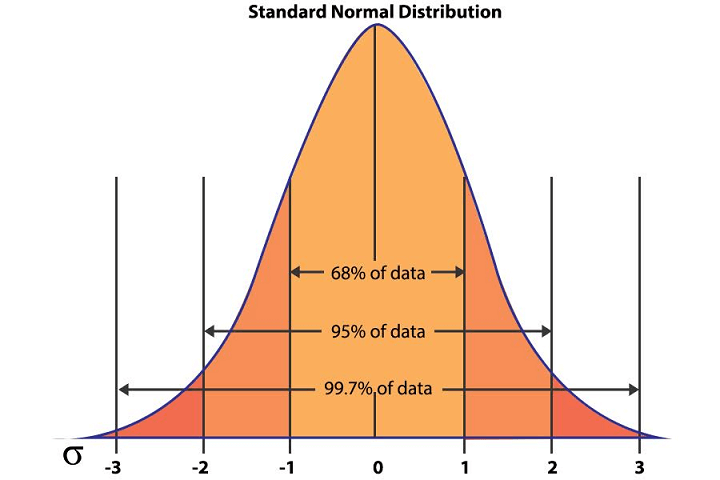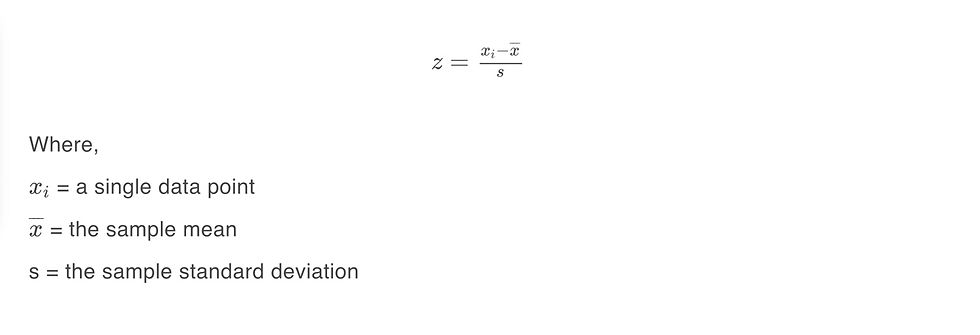top of page

# Standard deviation in Finance and Trading.As we can see, by its very construction, the variance is in the square of the original unit. This means that if we are dealing with distances in kilometers, the unit of variance would be in square kilometers.

Now, square kilometers may be easy to visualize as a unit, but what about year2year2 or IQ2IQ2, if we are working with the ages or IQs of a group? They are harder to interpret.

Hence, it makes sense to use a measure that can be comparable to the data on the same scale/units, like the standard deviation.

Standard deviation is calculated as the square root of variance. It has the same unit as our data and this makes it easy to use and interpret.

For example, consider a scenario where we are looking at a dataset of the heights of residents of a neighborhood. Assume that the heights are normally distributed with a mean of 165 cm and a standard deviation of 5 cm.

We know that for a normal distribution,

• 68% of the data points fall within one standard deviation,

• 95% within two standard deviations, and

• 99.7% fall within three standard deviations from the mean.Standard Normal Distribution (Image Source: Standard Normal Distribution)

Thus, we can conclude that the height of almost 68% of the residents would lie between one standard deviation from the mean, i.e., between 160 cm (mean – sd) and 170 cm (mean + sd). You can read more about normal distribution here.

### Standard deviation for sample data - Bessel's correction

When calculating the standard deviation of a population, we use the formula discussed above. However, we modify it slightly when dealing with a sample instead.

This is because the sample is much smaller compared to the entire population. In order to account for differences in a randomly selected sample and the entire population, we ‘unbias’ the calculation by using '(n-1)' instead of 'n' in the denominator of equation 1. This is referred to as Bessel's correction.

Thus, we use the following formula to calculate the sample standard deviation (s).### Yahoo Finance

One of the first sources from which you can get historical daily price-volume stock market data is Yahoo finance. You can use pandas_datareader or yfinance module to get the data and then can download or store it in a CSV file by using pandas.to_csv method.

If yfinance is not installed on your computer, then run the below line of code from your Jupyter Notebook to install yfinance.

`!pipinstallyfinance`

### Standard deviation is a measure of volatility

In trading and finance, it is important to quantify the volatility of an asset. An asset’s volatility, unlike its return or price, is an unobserved variable.

The standard deviation has a special significance in risk management and performance analysis as it is often used as a proxy for the volatility of a security. For example, the well-established blue-chip securities have a lower standard deviation in their returns compared to that of small-cap stocks.

On the other hand, assets like cryptocurrency have a higher standard deviation, as their returns vary widely from their mean.

In the next section, we will learn to compute the annualized volatility of stocks in Python.

### Computing annualized volatility of stocks using Python

Let us now compute and compare the annualized volatility for two Indian stocks namely, ITC and Reliance. We begin with fetching the end of day close price data using the yfinance library for a period of the last 5 years:

```import yfinance as yf
import warningswarnings.filterwarnings('ignore')

# Download the data for ITC and RELIANCE stocks using yahoo finance library

# Taking a peek at the fetched dataitc_df.tail()```
```Date           Adj Close
2021-10-19     245.949997
2021-10-20     246.600006
2021-10-21     244.699997
2021-10-22     236.600006
2021-10-25     234.350006```
`reliance_df.tail()`
```Date          Adj Close
2021-10-19   2731.850098
2021-10-20   2700.399902
2021-10-21   2622.500000
2021-10-22   2627.399902
2021-10-25   2607.300049```

Below, we calculate the daily returns using the pct_change() method and the standard deviation of those returns using the std() method to get the daily volatilities of the two stocks:

```# Compute the returns of the two stocksitc_df['Returns']

```# Compute the standard deviation of the returns using the pandas std() method

daily_sd_itc=itc_df['Returns'].std()daily_sd_rel=reliance_df['Returns'].std()```
`reliance_df.dropna(inplace=True)reliance_df.head()`
```Date            Adj Close	 Returns
2016-10-26	508.709717	-0.006410
2016-10-27	506.127686	-0.005076
2016-10-28	509.144104	 0.005960
2016-11-01	507.237701	-0.003744
2016-11-02	494.086243	-0.025928```

In general, the volatility of assets is quoted in annual terms. So below, we convert the daily volatilities to annual volatilities by multiplying with the square root of 252 (the number of trading days in a year):

```import numpy as np
# Annualized standard deviation

annualized_sd_itc=daily_sd_itc*np.sqrt(252)
annualized_sd_rel=daily_sd_rel*np.sqrt(252)

print(f'The annualized standard deviation of the ITC stock daily returns is: {annualized_sd_itc*100:.2f}%')

print(f'The annualized standard deviation of the Reliance stock daily returns is: {annualized_sd_rel*100:.2f}%')```
```The annualized standard deviation of the ITC stock daily returns is: 27.39%

The annualized standard deviation of the Reliance stock daily returns is: 31.07%```

Now we will compute the standard deviation with Bessel's correction. To do this, we provide a ddof parameter to the Numpy std function. Here, ddof means 'Delta Degrees of Freedom'.

By default, Numpy uses ddof=0 for calculating standard deviation- this is the standard deviation of the population. For calculating the standard deviation of a sample, we give ddof=1, so that in the formula, (n−1) is used as the divisor. Below, we do the same:

```# Compute the standard deviation with Bessel's correction

daily_sd_itc_b=itc_df['Returns'].std(ddof=1)daily_sd_rel_b=reliance_df['Returns'].std(ddof=1)

# Annualized standard deviation with Bessel's correction

annualized_sd_itc_b=daily_sd_itc_b*np.sqrt(252)
annualized_sd_rel_b=daily_sd_rel_b*np.sqrt(252)

print(f'The annualized standard deviation of the ITC stock daily returns with Bessel\'s correction is: {annualized_sd_itc_b*100:.2f}%')

print(f'The annualized standard deviation of the Reliance stock daily returns with Bessel\'s correction is: {annualized_sd_rel_b*100:.2f}%')```
```The annualized standard deviation of the ITC stock daily returns with Bessel's correction is: 27.39%

The annualized standard deviation of the Reliance stock daily returns with Bessel's correction is: 31.07%

```

Thus, we can observe that, as the sample size is very large, Bessel's correction does not have much impact on the obtained values of standard deviation. In addition, based on the given data, we can say that the Reliance stock is more volatile compared to the ITC stock.

Note: The purpose of this illustration is to show how standard deviation is used in the context of the financial markets, in a highly simplified manner. There are factors such as rolling statistics (outside the scope of this write-up) that should be explored when using these concepts in strategy implementation.

### The z-score

Z-score is a metric that tells us how many standard deviations away a particular data point is from the mean. It can be negative or positive. A positive z-score, like 1, indicates that the data point lies one standard deviation above the mean, and a negative z-score, like -2, implies that the data point lies two standard deviations below the mean.

In financial terms, when calculating the z-score on the returns of an asset, a higher value of z-score (either positive or negative) means that the return of the security differs significantly from its mean value. So, the z-score tells us how well the data point conforms to the norm.

Usually, if the absolute value of a z score of a data point is very high (say, more than 3), it indicates that the data point is quite different from the other data points.

We use standard deviation to calculate the z-score using the following formula in case we have sample data:Below we calculate and plot the z-scores for the ITC stock returns using the above formula in Python:

`itc_df['z-score'] = (itc_df['Returns'] -itc_df['Returns'].mean())/itc_df['Returns'].std(ddof=1)`
```import matplotlib.pyplot as plt
itc_df['z-score'].plot(figsize=(20,10));
plt.axhline(-3, color='r')
plt.title('Z-scores for ITC stock returns')
plt.show();```Z-scores for ITC stock returns

From the above figure, we observe that around March of 2020, the ITC stock returns had a z-score reaching below -3 several times, indicating that the returns were more than 3 standard deviations below the mean for the given data sample. As we know that this was during the sell-off triggered by the COVID pandemic.

Also, one can use the zscore function from the scipy.stats module to calculate the z-scores as follows:

```# Computing z-scores in python using scipy.stats module

import scipy.stats as stats
reliance_df['Returns_zscore'] =stats.zscore(reliance_df['Returns'])
reliance_df.tail()```
```Date		 Adj Close	 Returns     Returns_zscore
2021-10-19	2731.850098	 0.008956	 0.380491
2021-10-20	2700.399902	-0.011512	-0.665617
2021-10-21	2622.500000	-0.028848	-1.551575
2021-10-22	2627.399902	 0.001868	 0.018247
2021-10-25	2607.300049	-0.007650	-0.46822```

### Value at Risk

Value at Risk (VaR) is an important financial risk management metric that quantifies the maximum loss that can be realized in a given time with a given level of confidence/probability for a given strategy, portfolio, or trading desk.

It can be computed in three ways, one of which is the variance-covariance method. In this method, we assume that the returns are normally distributed for the lookback period.

The idea is simple. We calculate the z-score of the returns of the strategy based on the confidence level we want and then multiply it with the standard deviation to get the VaR. To get the VaR in dollar terms, we can multiply it with the investment in the strategy.

For example, if we want the 95% confidence VaR, we are essentially finding the cut-off point for the worst 5% of the losses from the returns distribution. If we assume that the stock returns are normally distributed, then their z-scores will have a standard normal distribution. So, the cut-off point for the worst 5% returns is -1.64:VaR z-score cut-off point

Thus the 1-year 95% VaR of a simple strategy of investing in the ITC stock is given by:

VaR = (−1.64) ∗ (s) ∗ investment

where, s is the annualized standard deviation of the ITC stocks.

```#1 year 95% VaR calculation for ITC stock:

from scipy.stats import norm

initial_investment=100000
annual_standard_deviation=annualized_sd_itc
confidence_level=.95

# using the norm.ppf (percent point function), calculate the value where 95% our data lies
z_score_cut_off=norm.ppf(1-confidence_level, 0, 1)

```
`z_score_cut_off`
`-1.6448536269514722`
```VaR = z_score_cut_off * annual_standard_deviation * initial_investment
VaR```
`-45045.34407051503`

Thus, we can say that the maximum loss that can be realized in 1 year with 95% confidence is INR 45045. Of course, this was calculated under the assumption that ITC stock returns follow a normal distribution.

## Confidence intervals

Another common use case for standard deviation is in computing the confidence intervals.

In general, when we work with data, we assume that the population from which the data has been generated follows a certain distribution, and the population parameters for that distribution are not known. These population parameters have to be estimated using the sample.

For example, the mean daily return of the ITC stock is a population parameter, which we try to estimate using the sample mean. This gives us a point estimate. However, financial market forecasts are probabilistic, and hence, it would make more sense to work with an interval estimate rather than a point estimate.

A confidence interval gives a probable estimated range within which the value of the population parameter may lie. Assuming the data to be normally distributed, we can use the empirical rule to describe the percentage of data that falls within 1, 2, and 3 standard deviations from the mean.

1. About 68% of the values lie between -1 and +1 standard deviation from the mean.

2. About 95% of the values lie within two standard deviations from the mean.

3. About 99.7% of the values lie within three standard deviations from the mean.

```# Compute the sample mean of the ITC returns

daily_mean_itc=itc_df['Returns'].mean()

#Compute 95% confidence interval for the ITC returns
ci_95_itc_upper=daily_mean_itc+2*daily_sd_itc_b
ci_95_itc_lower=daily_mean_itc-2*daily_sd_itc_b

print(f'The 95% confidence interval of the ITC stock daily returns is: [{ci_95_itc_lower:.2f},{ci_95_itc_upper:.2f}]')```
`The 95% confidence interval of the ITC stock daily returns is: [-0.03,0.03]`

Thus, we can say that based on the data we have, the mean daily return of the ITC stock is 95% likely to be a value between -3% and +3% (assuming the ITC stock returns are normally distributed).

bottom of page